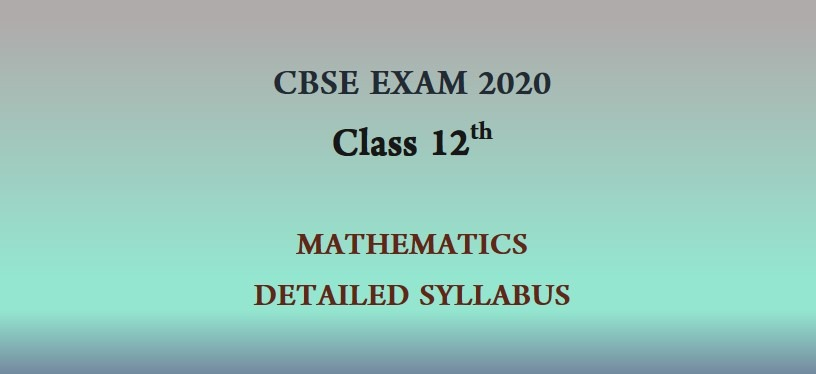Most Affordable JEE | NEET | 8,9,10 Preparation by Kota's Top IITian Doctor FacultiesHere in this article the students can find the detailed CBSE Class 12 Mathematics Syllabus 2019-20. Each chapter is described topic wise. Students should follow this and prepare according to it for the exams. Mathematics
 UNIT TOPICS Unit-I: Sets and Functions Sets ·Sets and their representations. Empty set.Finite and Infinite sets Equal sets.Subsets.Subsets of a set  of  real  numbers  especially  intervals  (with  notations). Power  set.  Universal  set. Venn diagrams. Union and Intersection of sets.Difference of sets Complement of a set. Properties of Complement. Relations & Functions ·         Ordered pairs.Cartesian product of sets.Number of elements in the Cartesian product of two finite sets. ·         Cartesian product of the set of reals with itself (upto R x R x R). ·         Definition of relation, pictorial diagrams,  domain,  co-domain  and  range  of  a  relation. ·         Function  as  a  special  type  of  relation. ·         Pictorial  representation  of  a  function,  domain,  co-domain  and  range  of  a function. ·         Real  valued functions, domain and range of these functions, constant, identity, polynomial, rational, modulus, signum,  exponential,  logarithmic  and  greatest  integer  functions,  with  their  graphs. ·          Sum, difference, product and quotients of functions. Trigonometric Functions ·         Positive  and  negative  angles.Measuring  angles in  radians and in degrees and conversion from one measure to another. ·         Definition of trigonometric functions with the help of unit circle. ·         Truth of the  identity sin2x + cos2x = 1, for all x. ·         Signs of  trigonometric functions. ·         Domain  and range  of ·         trigonometric functions and their graphs. ·         Expressing sin (x±y) and cos (x±y) in terms of sinx, siny, cosx&cosy and their simple applications. Unit-II: Algebra Principle of Mathematical Induction  ·         Process of the proof by induction, motivating the application of the method by looking at natural numbers  as  the  least  inductive  subset  of  real  numbers.The  principle  of  mathematical  induction and simple applications Complex Numbers and Quadratic Equations Need  for  complex  numbers,  especially √−1 ,  to  be  motivated  by  inability  to  solve  some  of  the Quardratic   equations.   Algebraic   properties   of   complex   numbers.Argand   plane   and   polar representation  of  complex  numbers.Statement  of  Fundamental  Theorem of Algebra,  solution  of quadratic  equations  (with  real  coefficients)  in  the  complex  number  system.Square  root  of  a complex number.   Linear Inequalities ·         Linear   inequalities.Algebraic   solutions   of   linear   inequalities   in   one   variable   and   their representation    on    the    number    line.Graphical    solution    of    linear    inequalities    in    two variables.Graphical method of finding a solution of system of linear inequalities in two variables   Permutations and Combinations ·Fundamental  principle  of  counting.Factorialn.  (n!)  Permutations  and  combinations,  derivation of Formulae for n h and their connections, simple applications. Binomial Theorem  History,  statement  and  proof  of  the  binomial  theorem  for  positive  integral  indices.Pascal's triangle, General and middle term in binomial expansion, simple applications.   Sequence and Series ·         Sequence  and  Series.  Arithmetic  Progression  (A.  P.).  Arithmetic  Mean  (A.M.)  Geometric Progression (G.P.), general term of a G.P., sum of n terms of a G.P., infinite G.P. and its sum, geometric  mean  (G.M.),  relation  between  A.M.  and  G.M Unit-III: Coordinate Geometry Straight Lines ·         Brief  recall  of  two  dimensional  geometry  from  earlier  classes.  Shifting  of  origin.  Slope  of a  line and angle between two lines. Various forms of equations of a line: parallel to axis, point -slope form, slope-intercept form, two-point form, intercept form and normal form. General equation of a line.Equation of family of lines passing through the point of intersection of two lines.Distance of a point from a line.   Conic Sections  ·         Sections  of  a  cone:  circles,  ellipse,  parabola,  hyperbola,  a  point,  a  straight  line  and  a  pair  of intersecting  lines  as  a  degenerated  case  of  a  conic  section.  Standard  equations  and  simple properties of parabola, ellipse and hyperbola.Standard equation of a circle.   Introduction to Three-dimensional Geometry ·         Coordinate  axes  and  coordinate  planes  in  three  dimensions.  Coordinates  of  a  point.  Distance between two points and section formula. Unit-IV: Calculus Limits and Derivatives   ·         Derivative    introduced    as    rate    of    change    both    as    that    of    distance    function    and geometrically.Intuitive  idea  of  limit.Limits  of  polynomials  and  rational  functions  trigonometric, exponential  and  logarithmic functions.Definition  of  derivative  relate  it to scope  of  tangent  of  the curve, derivative of sum, difference, product and quotient of functions. Derivatives of polynomial and trigonometric functions. Unit-V: Mathematical Reasoning Mathematical Reasoning ·         Mathematically   acceptable   statements.   Connecting   words/   phrases   -   consolidating   the understanding of "if and only if (necessary and sufficient) condition", "implies", "and/or", "implied by", "and", "or", "there exists" and their use through variety of examples related to real life and Mathematics.  Validating  the  statements  involving  the  connecting  words,  difference  among contradiction, converse and contra positive. Unit-VI: Statistics and Probability Statistics ·         Measures   of   Dispersion:   Range,   Mean   deviation,   variance   and   standard   deviation   of un grouped/grouped  data.  Analysis  of  frequency  distributions  with  equal  means  but  different variances.   Probability      ·         Random  experiments;  outcomes,  sample  spaces  (set  representation).  Events;  occurrence  of events, ‘not’, ‘and’ and ‘or’ events, exhaustive events, mutually exclusive events, Axiomatic (set theoretic)  probability,  connections with  other  theories  of  earlier  classes. Probability  of  an  event, probability of ‘not’, ‘and’ and ‘or’ events.
DOWNLOAD eSaral APP for JEE & NEET Preparation. Get Free video lectures, tests, practice questions designed by Top IITian Faculties of Kota.
Class 12 PHYSICS Complete Notes

Class 12 CHEMISTRY Complete Notes

Class 12 MATHEMATICS Complete Notes`